MathematicsSolving PDE Problems

This section describes:

Example: A Single PDE

This example illustrates the straightforward formulation, solution, and plotting of the solution of a single PDE

•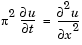This equation holds on an interval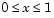for times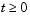. At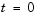, the solution satisfies the initial condition

•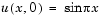At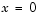and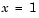, the solution satisfies the boundary conditions

•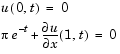Note    The demo `pdex1` contains the complete code for this example. The demo uses subfunctions to place all functions it requires in a single M-file. To run the demo type `pdex1` at the command line. See PDE Solver Basic Syntax for more information.

1. Rewrite the PDE. Write the PDE in the form
•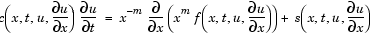1. This is the form shown in Equation 5-3 and expected by `pdepe`. See Introduction to PDE Problems for more information. For this example, the resulting equation is

•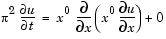1. with parameter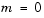and the terms

•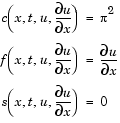1. Code the PDE. Once you rewrite the PDE in the form shown above (Equation 5-3) and identify the terms, you can code the PDE in a function that `pdepe` can use. The function must be of the form
• ``````[c,f,s] = pdefun(x,t,u,dudx)
``````
1. where `c`, `f`, and `s` correspond to the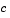,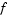, and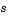terms. The code below computes `c`, `f`, and `s` for the example problem.

• ```function [c,f,s] = pdex1pde(x,t,u,DuDx)
c = pi^2;
f = DuDx;
s = 0;
```
1. Code the initial conditions function. You must code the initial conditions in a function of the form
• ``````u = icfun(x)
``````
1. The code below represents the initial conditions in the function `pdex1ic`.

• ```function u0 = pdex1ic(x)
u0 = sin(pi*x);
```
1. Code the boundary conditions function. You must also code the boundary conditions in a function of the form
• ``````[pl,ql,pr,qr] = bcfun(xl,ul,xr,ur,t)
``````
1. The boundary conditions, written in the same form as Equation 5-5, are

•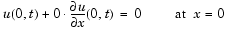1. and

•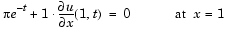1. The code below evaluates the components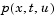and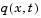of the boundary conditions in the function `pdex1bc`.

• ```function [pl,ql,pr,qr] = pdex1bc(xl,ul,xr,ur,t)
pl = ul;
ql = 0;
pr = pi * exp(-t);
qr = 1;
```

In the function `pdex1bc`, `pl` and `ql` correspond to the left boundary conditions (), and `pr` and `qr` correspond to the right boundary condition ().

1. Select mesh points for the solution. Before you use the MATLAB PDE solver, you need to specify the mesh points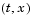at which you want `pdepe` to evaluate the solution. Specify the points as vectors `t` and `x`.
1. The vectors `t` and `x` play different roles in the solver (see MATLAB Partial Differential Equation Solver). In particular, the cost and the accuracy of the solution depend strongly on the length of the vector `x`. However, the computation is much less sensitive to the values in the vector `t`.

This example requests the solution on the mesh produced by 20 equally spaced points from the spatial interval [0,1] and five values of `t` from the time interval [0,2].

• ```x = linspace(0,1,20);
t = linspace(0,2,5);
```
1. Apply the PDE solver. The example calls `pdepe` with `m = 0`, the functions pdex1pde, pdex1ic, and pdex1bc, and the mesh defined by x and t at which `pdepe` is to evaluate the solution. The `pdepe` function returns the numerical solution in a three-dimensional array `sol`, where `sol(i,j,k)` approximates the `k`th component of the solution,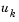, evaluated at `t(i)` and `x(j)`.
• ```m = 0;
sol = pdepe(m,@pdex1pde,@pdex1ic,@pdex1bc,x,t);
```
1. This example uses `@` to pass `pdex1pde`, `pdex1ic`, and `pdex1bc `as function handles to `pdepe`.

 Note    See the `function_handle` (@), `func2str`, and `str2func` reference pages, and the Function Handles chapter of "Programming and Data Types" in the MATLAB documentation for information about function handles.

1. View the results. Complete the example by displaying the results:
1. Extract and display the first solution component. In this example, the solution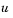has only one component, but for illustrative purposes, the example "extracts" it from the three-dimensional array. The surface plot shows the behavior of the solution.
• ```    u = sol(:,:,1);

surf(x,t,u)
title('Numerical solution computed with 20 mesh points')
xlabel('Distance x')
ylabel('Time t')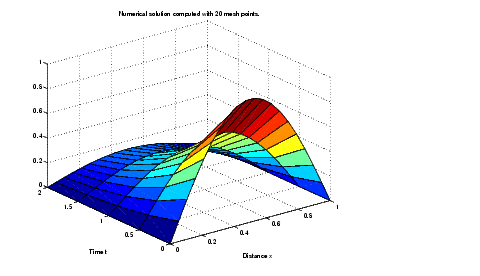```
2. Display a solution profile at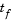, the final value of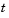. In this example,```=  ```
3. `2`. See Evaluating the Solution at Specific Points for more information.
• ```    figure
plot(x,u(end,:))
title('Solution at t = 2')
xlabel('Distance x')
ylabel('u(x,2)')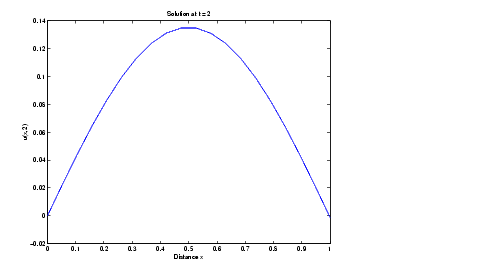```MATLAB Partial Differential Equation Solver Evaluating the Solution at Specific Points© 1994-2005 The MathWorks, Inc.